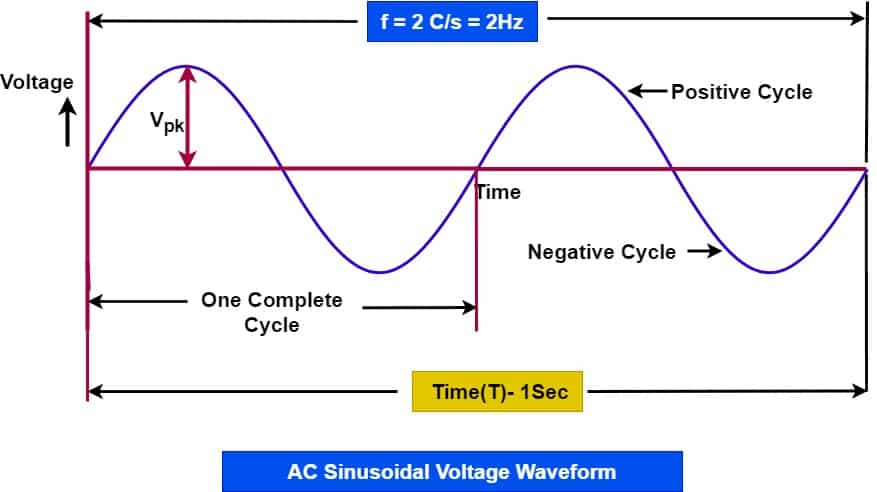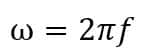# What is frequency?-Definition and Types of Frequency

Definition of frequency-The number of periodic oscillation completed in one unit time is called the frequency.The oscillatory signal completes the number of repeating events in a definite  time  period. The frequency is used for defining rotation per cycles of wave, oscillation and rotation.The SI unit of frequency is Hertz (Hz).Hertz is named after German physicist Heinrich Hertz, first to broadcast and receive radio waves. Radio waves travel at one cycle per second (1 Hz). One oscillation means one complete cycle. Therefore, we can denote the frequency by cycles/second or revolution/second which is equal to 1 Hz.  The wave has frequency 5 HZ, it means the wave completes  5 cycles in one second.

Example

A rotor of an induction motor rotates 1500 rounds in one minute. What is the frequency of rotation.

No of cycles or rotation = 1500
Time  taken to complete 1500 rotation=  60 Minutes
= 60 X 60 =3600 Seconds
” Number of Cycles completed in one second is frequency”

Frequency = No. of Cycles/Time
= 1500/3600
= 0.41 rotation/cycle
f= 0.41 Hz

Frequency is widely used for electrical voltage and current in electrical AC circuit. In the case of electrical voltage and current,  the voltage and current waveform is sinusoidal, and a sine wave repeats  number of times a sine wave completes. A sinusoidal waveform completes one cycle going through a positive to negative half cycle. The frequency of voltage is 50 Hz in India and 60 Hz in US. Alternating voltage and current of  50 Hz frequency means sine wave complete 50 cycles in one second.

## Frequency of AC voltage and AC current

We can further understand the frequency of AC voltage and current with the help of sinusoidal waveform. The various terms used for alternating voltage and current are follows.

• Frequency Hertz (Hz)  – 1 Hz = 1 Cycle/second
• Time period(T)  – Time taken to complete one  cycle [T= 1/f ]
•  positive to negative cycle

If an alternating current or voltage  has frequency of 3 Hz (see diagram below), that indicates its waveform repeats 2 times in 1 second.The more number of cycles per second means the more frequency.

### Range of Frequency for Electrical Equipment

The various electrical equipment functions on different frequency. The frequency used for equipment depends on the type of application. Frequency range of some of the electrical equipment are  given below.

 Type of Equipment Frequency Range Power Line Frequency 50 Hz to 60 Hz Variable Frequency Drive( Carrier Frequency) 1 Khz to 20 KHz Audio Frequency Range 15 Hz to 20 KHz Very High Frequency 30 to 300 MHz Ultra High Frequency 300 to 3000 MHz Super High Frequency 3 to 30 GHz Extremely High Frequency 30 to 300 GHz Radio frequency 30-300 kHz. Low frequency 300 kHz to 3 megahertz (MHz). Medium frequency 3-30 MHz.

### Importance of Frequency for Electrical Equipment

The frequency of AC power is very important.We design the electrical circuits and equipment to operate at a fixed frequency. The circuit components can work reliably at the frequency for which circuit components are designed. The frequency must remain within the specified range 50 +/- 1.5 Hz. The performance of the electrical equipment drastically deteriorated with under and over frequency from the specified frequency range. For example an induction motor designed for 50 Hz, and if we feed more than 50 Hz frequency, then the speed of motor increase and correspondingly its torque decrease.

## Types of Frequency

The frequency is broadly classified into two categories.

1. Angular Frequency

The angular frequency  measures angular displacement per unit time.  Its units is degrees (or radians) per second.The unit of angular frequency is Hertz. The relation between the frequency and angular frequency is ;Where, ω – angular frequency in rad/second

If frequency is 50 Hz, then angular frequency is;
ω = 2πf = 2 x 3.14 x 50 =314 rad/second

We can represent the sinusoidal waveform as;

ν  = V0 Sinωt = V0 Sin 314t
Where,

ν  = Instantaneous value of sine wave
V0 = Peak Value of sine wave

2. Spatial Frequency

It shows the characteristic of any structure that is periodic cross position in space. SI unit of spatial frequency is cycles per meter.It is inversely proportional to the wavelength.